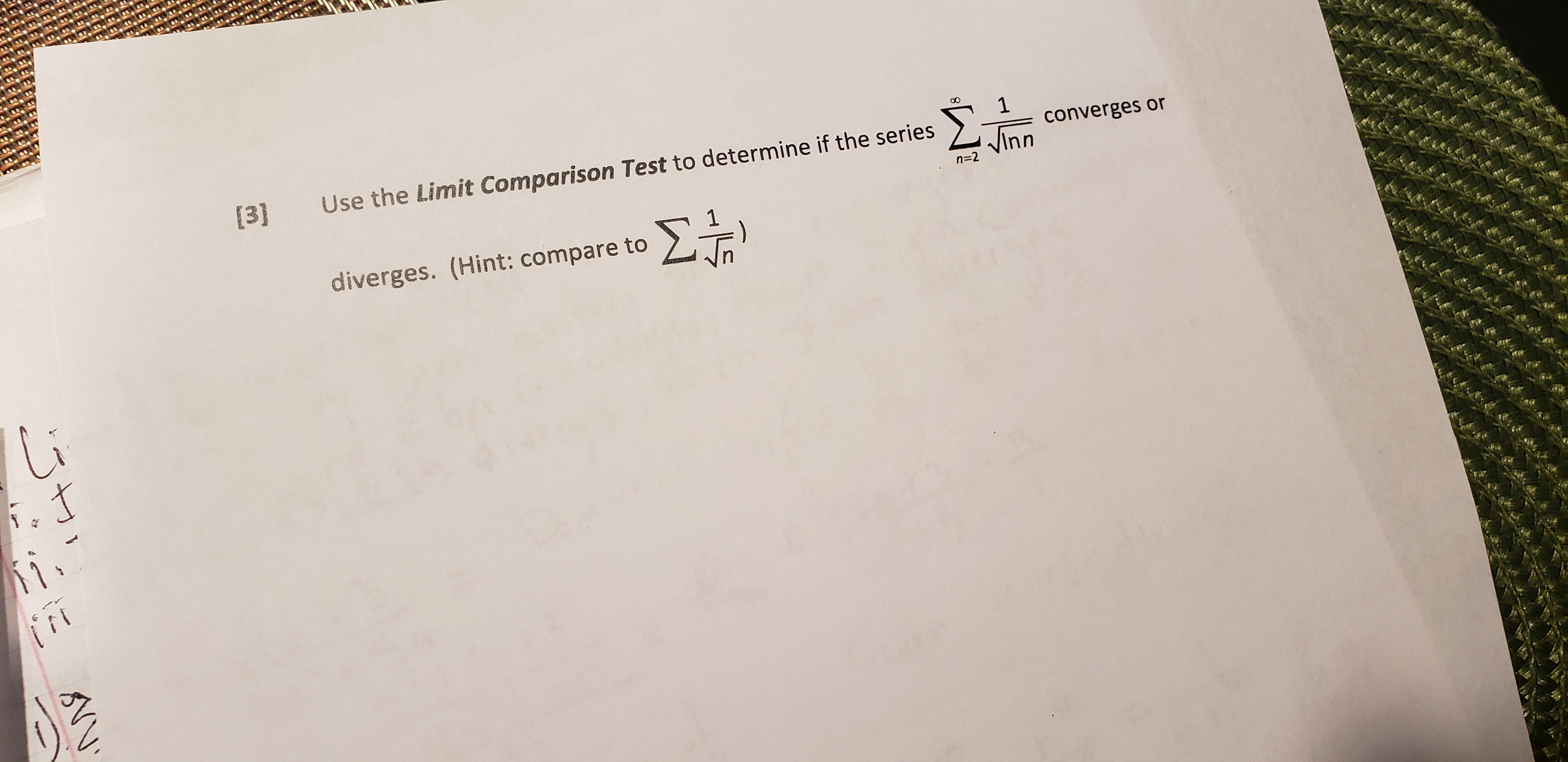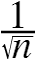# Use the Limit Comparison Test to determine if the seriesΣ.1converges orInnn=2Σ.diverges. (Hint: compare to.tKt

Question
19 viewshelp_outlineImage Transcriptionclose Use the Limit Comparison Test to determine if the series Σ. 1 converges or Inn n=2 Σ. diverges. (Hint: compare to .t Kt fullscreen
check_circle

Step 1

Consider the given series:

Step 2

Now, further

Since,

Step 3

It is known that, the series ∑is divergent by p-series test, since here p=1/2, which is less than 1, hence divergent.

Now, by limit comparison test: for two series an and bn such that an ≤ bn then if ∑an is divergent then so is ∑bn...

### Want to see the full answer?

See Solution

#### Want to see this answer and more?

Solutions are written by subject experts who are available 24/7. Questions are typically answered within 1 hour.*

See Solution
*Response times may vary by subject and question.
Tagged in

### Other